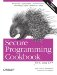# Computing the Sum and Mean of Elements in a Container

Problem

You want to compute the sum and mean of elements in a container of numbers.

Solution

You can use the accumulate function from the header to compute the sum, and then divide by the size to get the mean. Example 11-5 demonstrates this using a vector.

Example 11-5. Computing the sum and mean of a container

```#include
#include
#include

using namespace std;

int main( ) {
vector v;
v.push_back(1);
v.push_back(2);
v.push_back(3);
v.push_back(4);
int sum = accumulate(v.begin( ), v.end( ), 0);
double mean = double(sum) / v.size( );
cout << "sum = " << sum << endl;
cout << "count = " << v.size( ) << endl;
cout << "mean = " << mean << endl;
}```

The program in Example 11-5 produces the following output:

```sum = 10
count = 4
mean = 2.5```

Discussion

The accumulate function generally provides the most efficient and simplest method to find the sum of all the elements in a container.

Even though this recipe has a relatively simple solution, writing your own generic function to compute a mean is not so easy. Example 11-6 shows one way to write such a generic function:

Example 11-6. A generic function to compute the mean

```template
double computeMean(Iter_T first, Iter_T last) {
return static_cast(accumulate(first, last, 0.0))
/ distance(first, last);
}```

The computeMean function in Example 11-6 is sufficient for most purposes but it has one restriction: it doesn't work with input iterators such as istream_iterator.

## istream_iterator and ostream_iterator

The istream_iterator and ostream_iterator class templates are special-purpose iterators found in the the header that allow you to treat streams as single-pass containers.

The istream_iterator is an input iterator that wraps an input stream, such as cin or ifstream allowing it to be used as a parameter for many generic functions. The ostream_iterator is an output iterator that allows you to use output streams as if they are containers.

Using istream_iterator and ostream_iterator are good habits to get in, as they make it easier to write reusable code.

An istream_iterator allows only a single pass over the data, so you can call either accumulate or distance but once you call either function, the data is invalidated and any further attempts to iterate over the data will likely fail. Example 11-7 demonstrates how to write instead a more generic algorithm for computing the mean of a sequence of numbers in a single pass.

Example 11-7. A more generic function to compute the mean

```#include
#include
#include

using namespace std;

template
Value_T computeMean(Iter_T first, Iter_T last) {
if (first == last) throw domain_error("mean is undefined");
Value_T sum;
int cnt = 0;
while (first != last) {
sum += *first++;
++cnt;
}
return sum / cnt;
}

int main( ) {
cout << "please type in several integers separated by newlines" << endl;
cout << "and terminated by an EOF character (i.e., Ctrl-Z)" << endl;
double mean = computeMean(
istream_iterator(cin),
istream_iterator( ));
cout << "the mean is " << mean << endl;
}```

When writing generic code, you should always try to write for the most basic kind of iterator possible. This implies that whenever you can you should try to write generic algorithms that operate in a single pass over the input. By taking this approach, your generic code is not restricted only to containers, but can also be used with input iterators such as istream_iterator. As further motivation, single pass algorithms are often more efficient.

It may be surprising that I decided to write the computeMean function in Example 11-7 to require that the return type is passed as a template parameter as opposed to deducing it from the iterator type. This is because it is common for statistics to be computed and represented with a higher level of precision than the numbers in the container. For example, the code in Example 11-7 returns the mean of a set of integers as a double value.Secure Programming Cookbook for C and C++: Recipes for Cryptography, Authentication, Input Validation & More
ISBN: 0596003943
EAN: 2147483647
Year: 2006
Pages: 241

Similar book on Amazon